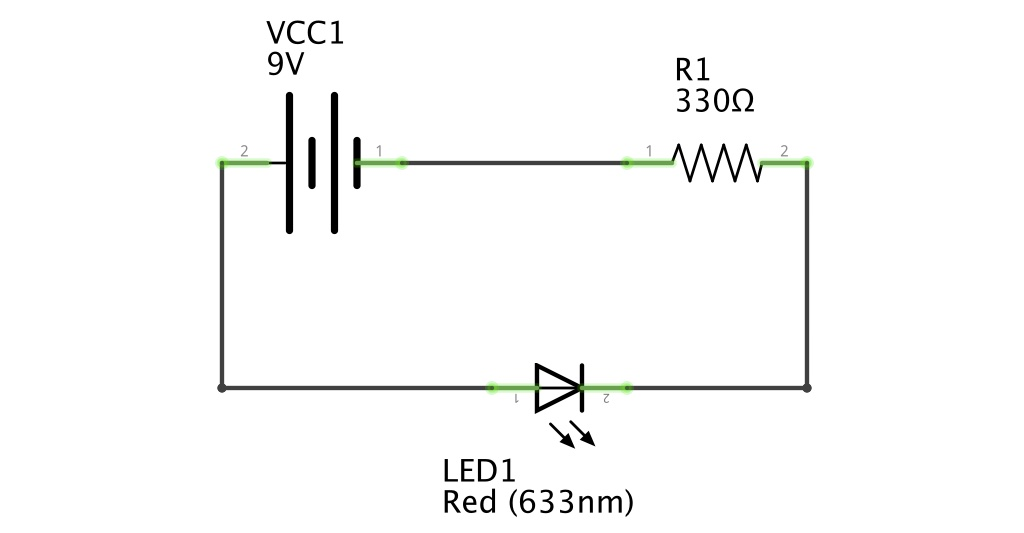## Ohm's LawEarlier on, we learned that a resistor provides resistance to the current flow. That means that current will leave the resistor with less energy than what it had before. If we calculate the potential difference between the two ends of the resistor, we will get the voltage of the resistor.

To do so, let's introduce you to Ohm's Law:

V (V) = R (Ω) * I (A)

What does this equation tell us? That the voltage - or potential difference - in the energy of the current depends on the resistance of the resistor and the amount of current.

So, the more current, the higher the difference and the higher the voltage.

So back to our circuit:

The current that an LED typically requires is around 0.02 Amps or 0.03 Amps. Anything much higher than 0.03 Amps will result in the LED blowing out. Anything less and the LED won't have enough energy to turn on.

R = 7V (voltage at the base of the resistor) / 0.02 A (current flowing through the circuit)

R = 350 Ohms (or the closest value available: 330Ω)